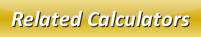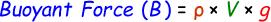# Buoyant Force Calculator

Buoyant Force is a type of force exerted on the object by fluid that acts upward due to fluid pressure and this force pushes the objects to be floated rather than to sink.

Formula to calculate buoyancy force is given by:where,
B = Buoyant force [N]
ρ = Fluid density [kg m-3]
V = Displaced volume [kg m-3]
g = Acceleration due to gravity [9.8 m/s2]

Use our below online buoyant force calculator by entering the given input values and click calculate to find the buoyant force.

 Fluid Density (ρ): [kg m-3] Displaced Volume (V): [kg m-3] Buoyant Force (B): [N]

Latest Calculator Release

Average Acceleration Calculator

Average acceleration is the object's change in speed for a specific given time period. ...

Free Fall Calculator

When an object falls into the ground due to planet's own gravitational force is known a...

Torque Calculator

Torque is nothing but a rotational force. In other words, the amount of force applied t...

Average Force Calculator

Average force can be explained as the amount of force exerted by the body moving at giv...

Angular Displacement Calculator

Angular displacement is the angle at which an object moves on a circular path. It is de...